# Find the Volume of Frustrums

In this worksheet, students will learn how to find the volume of frustums.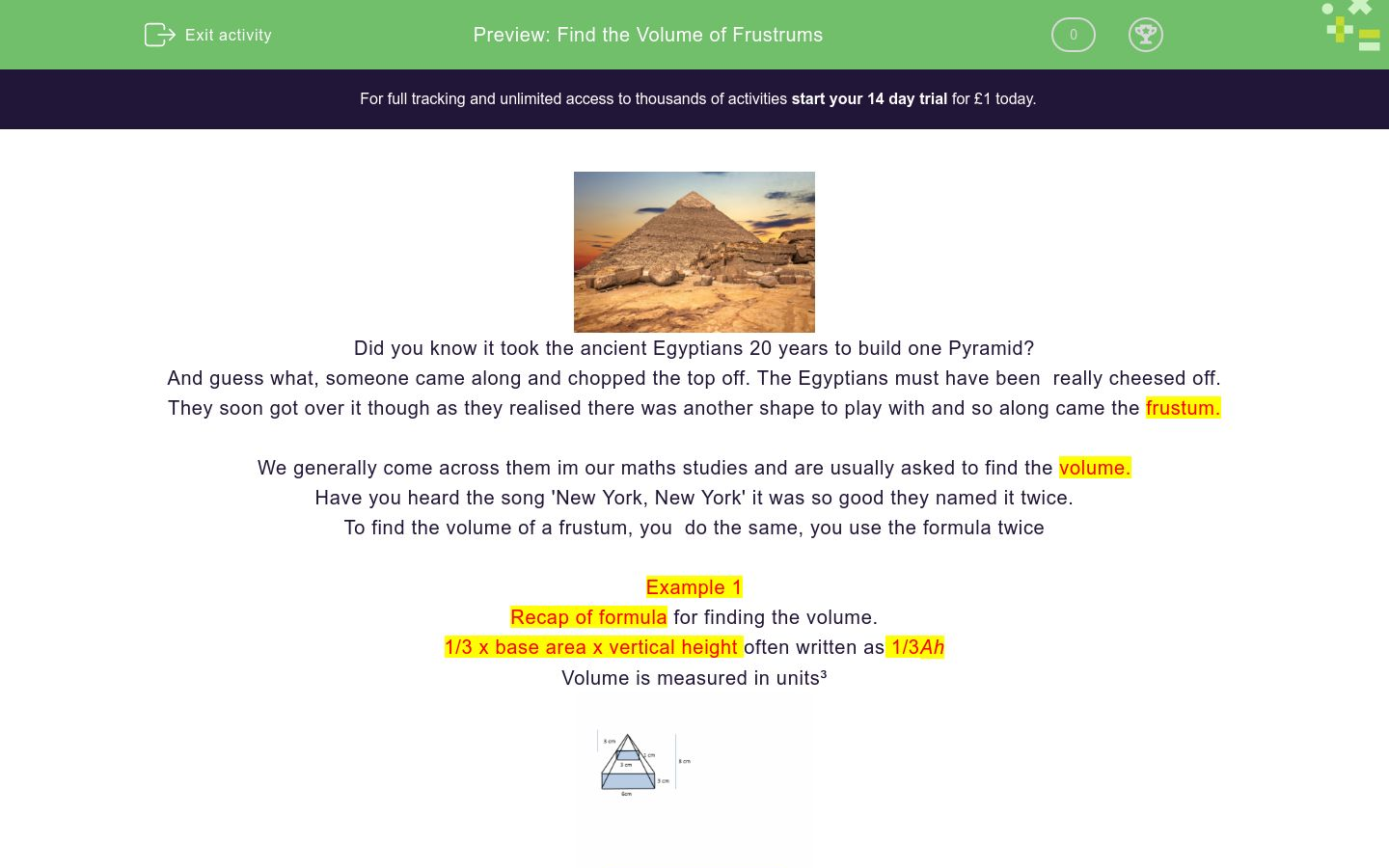Key stage:  KS 4

GCSE Subjects:   Maths

GCSE Boards:   AQA, Eduqas, Pearson Edexcel, OCR

Curriculum topic:   Geometry and Measures, Mensuration

Curriculum subtopic:   Mensuration and Calculation, Volume and Surface Area Calculations

Difficulty level:### QUESTION 1 of 10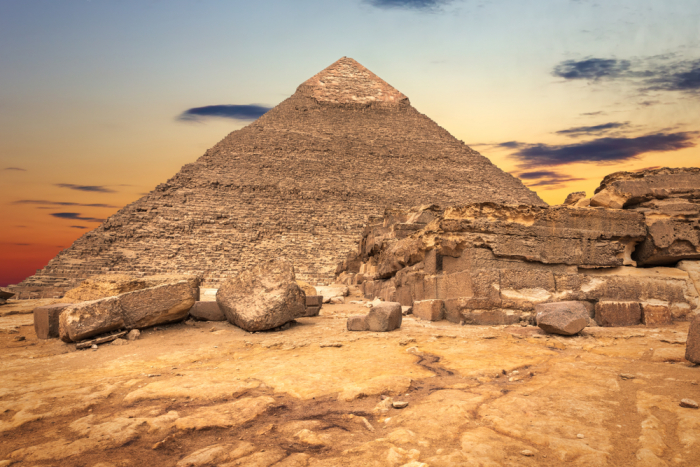Did you know it took the ancient Egyptians 20 years to build one Pyramid?

And guess what, someone came along and chopped the top off. The Egyptians must have been  really cheesed off.

They soon got over it though as they realised there was another shape to play with and so along came the frustum.

We generally come across them im our maths studies and are usually asked to find the volume.

Have you heard the song 'New York, New York' it was so good they named it twice.

To find the volume of a frustum, you  do the same, you use the formula twice

Example 1

Recap of formula for finding the volume.

1/3 x base area x vertical height often written as 1/3Ah

Volume is measured in units³Four simple steps

1. Calculate the volume of the square based Pyramid.

2. Calculate the volume of the smaller pyramid as shown

3.  Subtract the smaller volume from the larger one.

4.  Happy ancient Egyptians, happy you.

Base area of the larger pyramid = 6 x 3 = 18 cm²

Volume = 1/3 x 18 x 8 = 48 cm³

Base area of the smaller pyramid = 3 x 1 = 3 cm²

Volume = 1/3 x 3 x 3 = 3 cm³

Volume of the frustum = 48 - 3 = 45 cm³

Example 2Base area of large cone = Π x r² = 254.47 cm² (correct to 2 decimal places)

Volume of a full cone = 1/3 x 254.47 x 9 = 763.41 cm³

Base area of the smaller cone = π x r² = 63.62 cm²; (correct to 2 decimal places)

Volume of the smaller cone = 1/3 x 63.62 x 3 = 63.62 cm³

Volume of the frustum = 763.41 - 63.62 = 699.79 cm³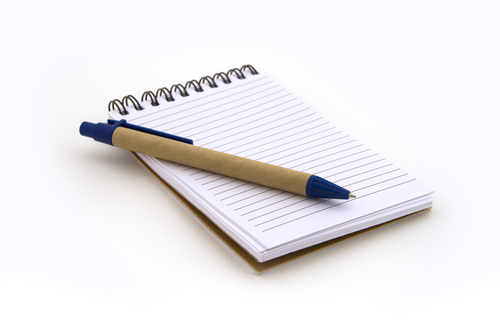Note

In the following activities the π button has been used on the calculator.

For 1/3 1÷ 3 used.

Round to two decimal places as you work through each part.Calculate the volume of the frustum.

 252 cm³ 14 cm³ 12 cm³ 240 cm³ 228 cm³ Volume of large pyramid Volume of small Pyramid Volume of the frustum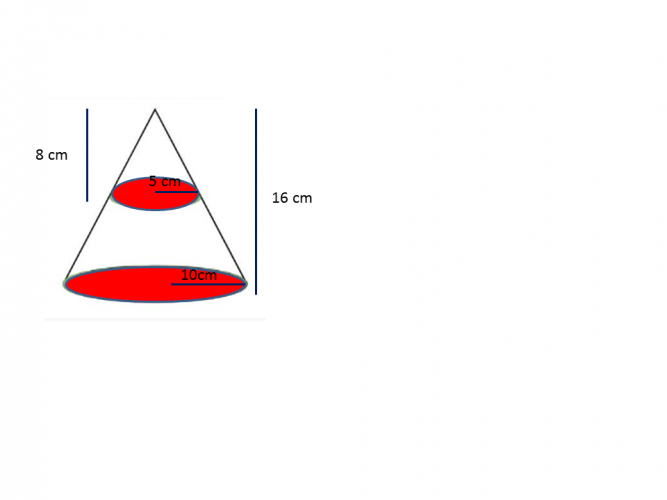1675.52 cm³ 1669.52 cm³ 200.44 cm³ 209.44 cm³ 1466.08 cm³ Volume of the larger cone Volume of the smaller cone Volume of the frustumThe volume of the frustum is 613.13 cm³ , 1944.03 cm³ , 1234.58 cm³ correct to 2 decimal places.The volume of the frustum is 613.13 cm³ , 1944.03 cm³ , 1234.58 cm³ correct to 2 decimal places.Calculate the volume of this frustum

84.00cm³

74.67 cm³

28 cm³

52.34 cm³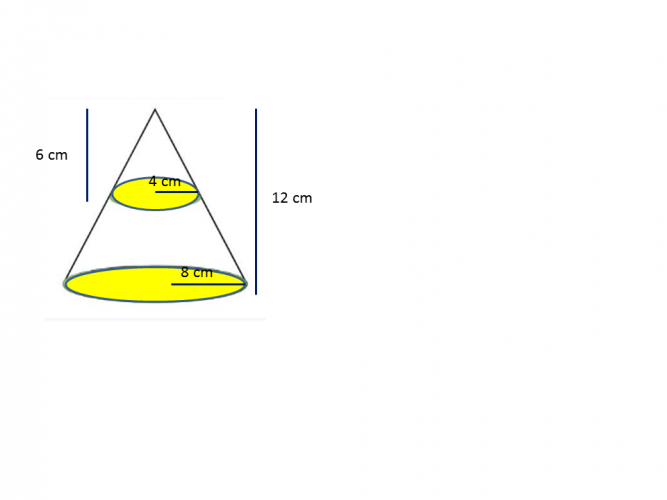Calculate the volume of the frustum.This paper cup is going to be made into a frustum.

The height of the cup is 15 cm.  The radius is 6 cm.

When the frustum is made the radius is half of the original and the height is one third of the original.

Calculate the volume of the frustum.

565.49 c³

608.37 cm³

612.61 cm³

518.37 cm³Calculate the volume of this frustum.

565.49 c³

608.37 cm³

612.61 cm³

518.37 cm³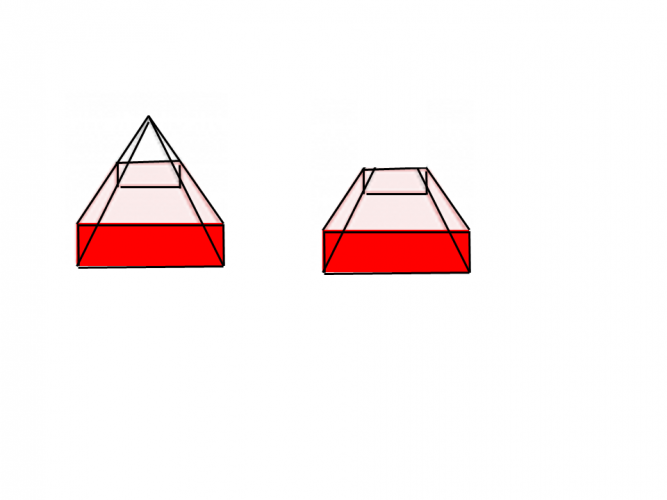The base area of the larger frustum is 40 cm&sup2;

The base area of the smaller frustum  18 cm&sup2;

The height of the large pyramid is 12 cm.

The height of the frustum is two thirds that of the large pyramid.

What is the volume of the frustum?

Write you answer in figures without the units.The dimensions of the pyramid are length 8 cm, width 6 cm and height 16 cm.

The smaller pyramid has dimensions one quarter of the size.

What is the volume of the frustum?

64 cm³

260 cm³

128 cm³

252 cm³

• Question 1Calculate the volume of the frustum.

 252 cm³ 14 cm³ 12 cm³ 240 cm³ 228 cm³ Volume of large pyramid Volume of small Pyramid Volume of the frustum
EDDIE SAYS
Quite often this type of question can look scary. Like anything in maths it is just a case of breaking things down to smaller chunks. Large Pyramid = 1/3 x 80 x 9 = 240 cm³ Small Pyramid = 1/3 x 12 x 3 = 12 cm³ Volume of frustum = 240 - 12 = 228 cm³ No scardy cats here.
• Question 21675.52 cm³ 1669.52 cm³ 200.44 cm³ 209.44 cm³ 1466.08 cm³ Volume of the larger cone Volume of the smaller cone Volume of the frustum
EDDIE SAYS
Can you see the value of breaking the task down. Don't try to do everything at once as you could get muddled. Larger cone = 1/3 x π x 10 x 10 x 16 = 1675.52 cm³ Small cone = 1/3 x π x 5 x 5 x 8 = 209.44 cm³ Volume of frustum = 1675.52 - 209.44 = 1466.08 cm³
• Question 3The volume of the frustum is 613.13 cm³ , 1944.03 cm³ , 1234.58 cm³ correct to 2 decimal places.
EDDIE SAYS
Did you spot it? You were given a diameter instead of a radius. Did you remember to halve the diameter first? Larger cone = 1/3 x π x 6 x 6 x 18 = 1678.58 cm³ Small cone = 1/3 x π x 2.5 x 2.5 x 10 = 265.45 cm³ Volume of frustum = 678.58 - 65.45 = 613.13 cm³
• Question 4EDDIE SAYS
How did ancient egyptians use the door bell to their pyramid? They just toot and come in. (ask someone older, if you need to) Larger Pyramid = 1/3 x 54 x 15 = 270 cm³ Small Pyramid = 1/3 x 6 x 5= 10 cm³ Volume of frustum = 270 - 10 = 260 cm³
• Question 5Calculate the volume of this frustum

74.67 cm³
EDDIE SAYS
Egypt is a very hot country. Did you know that the temperature inside a pyramid is a constant 20° celsius. Clever these Egyptians. Larger pyramid = 1/3 x 28 x 19 = 84 cm³ Small pyramid= 1/3 x 7 x 4 = 9.33 cm³ Volume of frustum = 84 - 9.33 = 74.67 cm³
• Question 6Calculate the volume of the frustum.

703.72
EDDIE SAYS
So when you go for an ice cream do you start of with a cone and end up with a frustum at some point? Never thought of that. Larger cone = 1/3 x π x 8 x 8 x 12 = 804.25cm³ Small cone = 1/3 x π x 4 x 4 x 6 = 100.53 cm³ Volume of frustum = 804.25 - 100.53 = 703.72 cm³
• Question 7This paper cup is going to be made into a frustum.

The height of the cup is 15 cm.  The radius is 6 cm.

When the frustum is made the radius is half of the original and the height is one third of the original.

Calculate the volume of the frustum.

518.37 cm³
EDDIE SAYS
Yes sometimes we have to calculate the answer from written information that is given. Write the numbers given on the diagram as it will look more familiar to you. Larger cone = 1/3 x π x 6 x 6 x 15 = 565.49 cm³ Small cone = 1/3 x π x 3 x 3 x 5 = 47.12 cm³ Volume of frustum = 565.49 - 47.12 = 518.37 cm³
• Question 8Calculate the volume of this frustum.

EDDIE SAYS
Are you getting the hang of this now? Larger cone = 1/3 x π x 6 x 6 x 12 = 452.38 cm³ Small cone = 1/3 x π x 3 x 3 x 5 = 47.12 cm³ Volume of frustum = 452.38 - 47.12 = 405.26 cm³
• Question 9The base area of the larger frustum is 40 cm&sup2;

The base area of the smaller frustum  18 cm&sup2;

The height of the large pyramid is 12 cm.

The height of the frustum is two thirds that of the large pyramid.

What is the volume of the frustum?

Write you answer in figures without the units.

112
EDDIE SAYS
Oh, thats what I like some of the work done already. Hey we don\'t have to work out the base area. The only thing we have to do before applying the formula is find the height of the frustum. As it is a two thirds that of the pyramid 12÷3 = 4 x 2 = 8 cm. Larger pyramid = 1/3x 40 x 12 = 160 cm³ Small pyramid = 1/3 x 18 x 8 = 48 cm³ Volume of frustum = 160 -48 = 112 cm³
• Question 10The dimensions of the pyramid are length 8 cm, width 6 cm and height 16 cm.

The smaller pyramid has dimensions one quarter of the size.

What is the volume of the frustum?

252 cm³
EDDIE SAYS
I hope you were not tempted to divide the answer to the first part by four. This sort of question is designed to get you to use information given before you arrive at the final answer. Larger pyramid = 1/3 x 8 x 6 x 16 = 256 cm³ Small pyramid = 1/3 x 2 x 1.5 x 4 = 4 cm³ Volume of frustum = 256 - 4 = 252 cm³
---- OR ----

Sign up for a £1 trial so you can track and measure your child's progress on this activity.

### What is EdPlace?

We're your National Curriculum aligned online education content provider helping each child succeed in English, maths and science from year 1 to GCSE. With an EdPlace account you’ll be able to track and measure progress, helping each child achieve their best. We build confidence and attainment by personalising each child’s learning at a level that suits them.

Get started